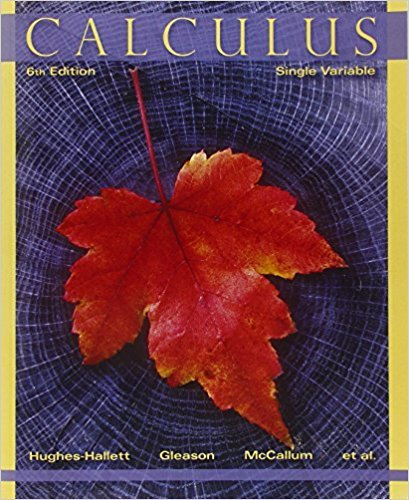×
×

# Solutions for Chapter 1.4: LOGARITHMIC FUNCTIONS## Full solutions for Calculus: Single Variable | 6th Edition

ISBN: 9780470888643Solutions for Chapter 1.4: LOGARITHMIC FUNCTIONS

Solutions for Chapter 1.4
4 5 0 283 Reviews
13
3
##### ISBN: 9780470888643

Calculus: Single Variable was written by and is associated to the ISBN: 9780470888643. This expansive textbook survival guide covers the following chapters and their solutions. This textbook survival guide was created for the textbook: Calculus: Single Variable , edition: 6. Chapter 1.4: LOGARITHMIC FUNCTIONS includes 68 full step-by-step solutions. Since 68 problems in chapter 1.4: LOGARITHMIC FUNCTIONS have been answered, more than 33453 students have viewed full step-by-step solutions from this chapter.

Key Calculus Terms and definitions covered in this textbook
• Additive identity for the complex numbers

0 + 0i is the complex number zero

• Convergence of a series

A series aqk=1 ak converges to a sum S if imn: q ank=1ak = S

• Decreasing on an interval

A function f is decreasing on an interval I if, for any two points in I, a positive change in x results in a negative change in ƒ(x)

• Definite integral

The definite integral of the function ƒ over [a,b] is Lbaƒ(x) dx = limn: q ani=1 ƒ(xi) ¢x provided the limit of the Riemann sums exists

• Directrix of a parabola, ellipse, or hyperbola

A line used to determine the conic

• Ellipsoid of revolution

A surface generated by rotating an ellipse about its major axis

• Finite sequence

A function whose domain is the first n positive integers for some fixed integer n.

• Focus, foci

See Ellipse, Hyperbola, Parabola.

• Heron’s formula

The area of ¢ABC with semiperimeter s is given by 2s1s - a21s - b21s - c2.

• Inverse properties

a + 1-a2 = 0, a # 1a

• NDER ƒ(a)

See Numerical derivative of ƒ at x = a.

• nth power of a

The number with n factors of a , where n is the exponent and a is the base.

• Parabola

The graph of a quadratic function, or the set of points in a plane that are equidistant from a fixed point (the focus) and a fixed line (the directrix).

• Periodic function

A function ƒ for which there is a positive number c such that for every value t in the domain of ƒ. The smallest such number c is the period of the function.

• Proportional

See Power function

• Quotient rule of logarithms

logb a R S b = logb R - logb S, R > 0, S > 0

The distance from a point on a circle (or a sphere) to the center of the circle (or the sphere).

• Solve by substitution

Method for solving systems of linear equations.

• y-intercept

A point that lies on both the graph and the y-axis.

• Zoom out

A procedure of a graphing utility used to view more of the coordinate plane (used, for example, to find theend behavior of a function).

×